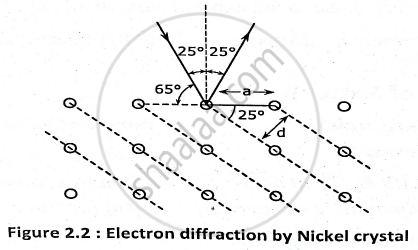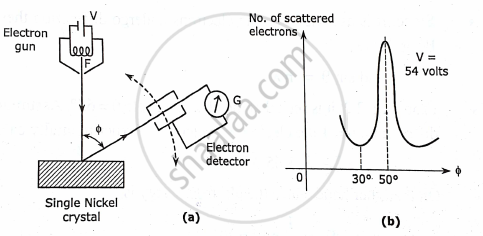Advertisement Remove all ads

# Describe with the Necessary Theory the Davisson and German Establishing Wave Nature of Electrons. - Applied Physics 1

Describe with the necessary theory the davisson and german establishing wave nature of electrons. Calculate the de-broglie wavelength of an alpha particle accelerating through a potential difference of 200 volts given :- mass of alpha
particle = 6.68×10-27kg.

Advertisement Remove all ads

#### Solution

According to de Broglie’s hypothesis, a beam of material particle must possess wave
like characteristics and should undergo phenomena like reflection , refraction, interference, diffraction and polarization as the ordinary light waves do. The first experimental verification of wave nature of atomic particles was provided by davisson and germer.

DAVISSON-GERMER EXPERIMENT.

In this experiment , from a hot filament F electrons are accelerated by a small voltage V to strike a target made of a single nickel crystal.

• The electrons are scattered off the crystal in all directions.

•  Help of an scattered electrons in all directions can be recorded with the help of an electron detector which can be rotated on a circular graduated scale.

•  For any accelerating voltage ,V the scattering curve shows a peak or maximum in a particular direction. It is found that for an accelerating voltage of 54 volts a very large number of electrons are scattered at a particular angle , ∅ = 50°.
•  It is assumed that the electron undergoes diffraction and the peak represents the first order spectrum at an angle of 50°.
The diffraction effects is explained as follows.

•  The atomic planes of the nickel crystal act like the ruling of a diffraction grating.
•  The interatomic distances of a nickel crystal is known to be a = 2.15 A°.
•  The interatomic spacing of a nickel crystal is d = 0.09 A°.• Since, it is assumed that the electrons undergo diffraction they must follow braggs law, 2dsinθ = nλ
•  It is seen that the glancing angle θ = 65°. Assuming the order of diffraction , n= 1 the electron wavelength is experimentally calculated as

λ_E = 1.65 A°.
• On the other hand since the electron energy is
E = 1/2mv^2 = eV
• The wavelength associated with an electron wave can be written as

λ_r = h/(sqrt(2mE)) = h/(sqrt(2meV)) = 12.25/sqrt(V)

• here the accelerating potential V = 54 volts for which the theoretical electron wavelength is found to be

λ_T = 1.67 A°.
• Which is very close to the experimental value , λ_E .
• This agreement confirms de Broglie hypothesis of matter waves.NUMERICAL:

Given data : - m_p = 6.68 × 10^-27 Kg , q_α = 4e = 6.4 xx 10^-19 c

V =200 volts.

Formul :- -1/2 mV^2 = q_α V , λ = h/(mV)
Solutions :-- 1/2 mV^2 = q_αV    , v = √ (2q_αV)/m

λ= h/(√2q_αmV)

= (6.63 xx 10^-34)/(√ 2 xx6.4 xx 10^-19 xx 6.68 xx 10^-27 xx 200

=0.507
Answer : de Broglie wavelength of an alpha particle
λ_α=0.507A°.

Concept: De Broglie Wavelength
Is there an error in this question or solution?
Advertisement Remove all ads

#### APPEARS IN

Advertisement Remove all ads
Advertisement Remove all ads
Share
Notifications

View all notifications

Forgot password?